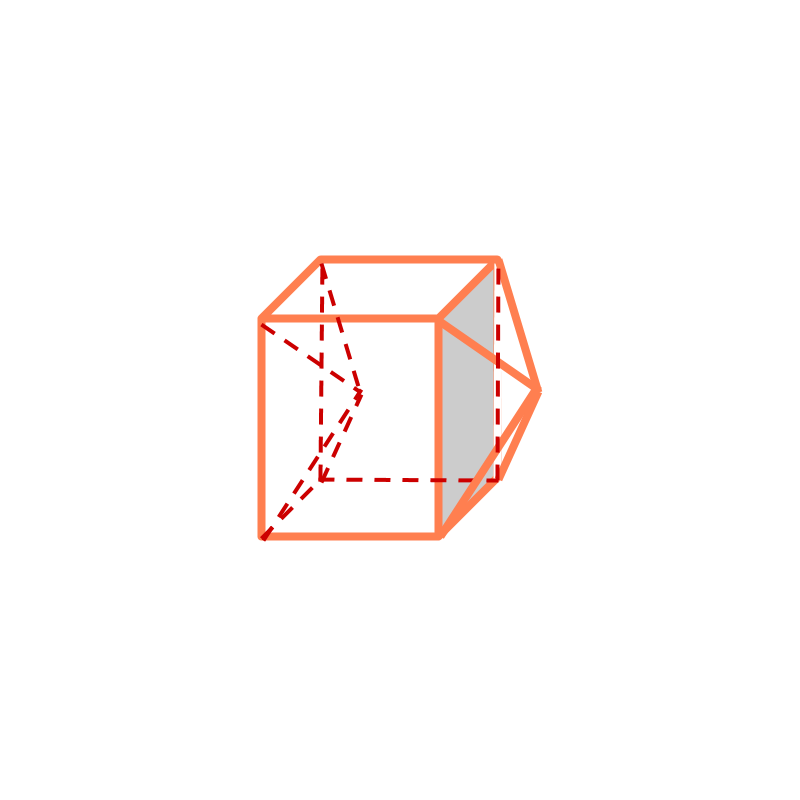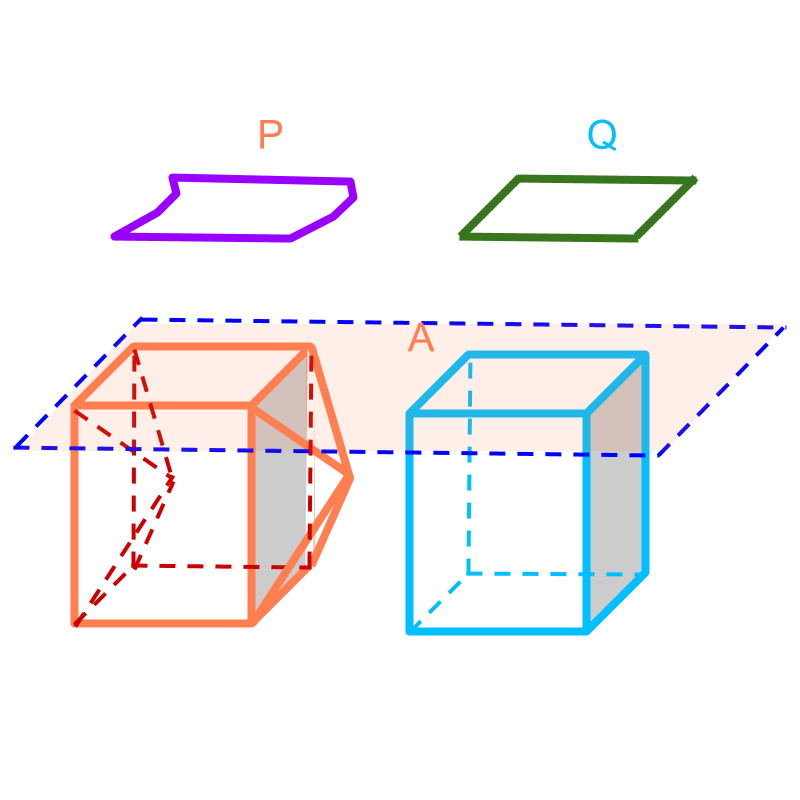maths > mensuration-high

Cavalieri's Principle (3D)

what you'll learn...

Overview

Cavalieri's Principle in 3D : For a given two solids, a plane intersecting the solids is considered. The area of the intersecting cross-sections $P$$P$ and $Q$$Q$ are considered.If the areas of intersecting cross-sections are equal for all planes parallel to the intersecting plane, then the volumes of the two solids are equal.

illustrating a problemConsider the solid given in the figure. It consists of four flat surfaces in the front, top, back, and bottom. And a pyramidal projection on the right. A similar pyramidal hollow-space on the left.

Spend some time to visualize this.

working out equivalenceConsider finding volume of the solid given in the figure. The solid is given in orange on the left. Another solid, a cuboid, is considered. The cuboid is shown in blue.

The two figures are aligned and a plane $A$$A$ intersecting them is drawn. The intersecting plane creates two plane-figures in the two solids at the cross-section. The two plane-figures are given as $P$$P$ and $Q$$Q$.

It is noted that the area of plane-figures are equal (by Cavalieri's principle in 2D).

As per Cavalieri's principle, if the areas of the cross-sections are equal for any plane parallel to the one shown, then the volume of the two solids are equal.

This implies that "the volume of the solid equals the volume of the cuboid".

summary

Cavalieri's Principle in 3D : For a given two solids, a plane intersecting the solids is considered. The area of the intersecting cross-sections $P$$P$ and $Q$$Q$ are considered.If the areas of intersecting cross-sections are equal for all planes parallel to the intersecting plane, then the volumes of the two solids are equal.

Outline